# 10 7 10 8 Volumeofof Pyramidsand and Cones

• Slides: 28
Download presentation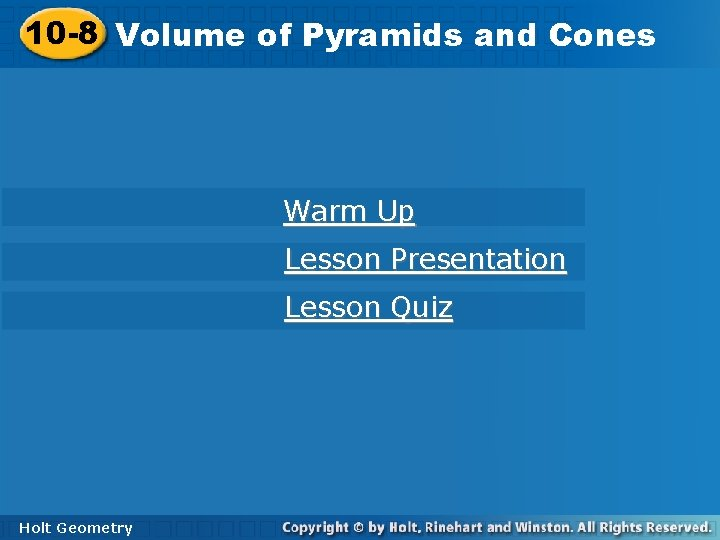10 -7 10 -8 Volumeofof. Pyramidsand and. Cones Warm Up Lesson Presentation Lesson Quiz Holt Geometry10 -8 Volume of Pyramids and Cones 10 -7 Warm Up Find the volume of each figure. Round to the nearest tenth, if necessary. 1. a square prism with base area 189 ft 2 and height 21 ft 3969 ft 3 2. a regular hexagonal prism with base edge length 24 m and height 10 m 14, 964. 9 m 3 3. a cylinder with diameter 16 in. and height 22 in. 4423. 4 in 3 Holt Geometry10 -8 Volume of Pyramids and Cones 10 -7 Objectives Learn and apply the formula for the volume of a pyramid. Learn and apply the formula for the volume of a cone. Holt Geometry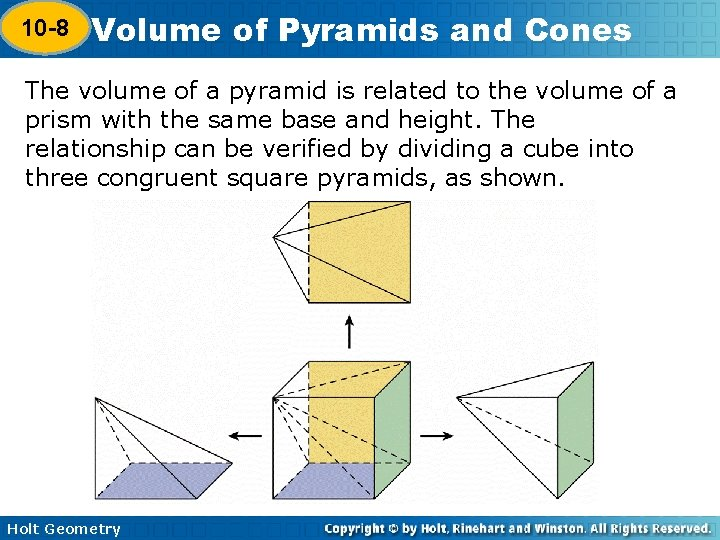10 -8 Volume of Pyramids and Cones 10 -7 The volume of a pyramid is related to the volume of a prism with the same base and height. The relationship can be verified by dividing a cube into three congruent square pyramids, as shown. Holt Geometry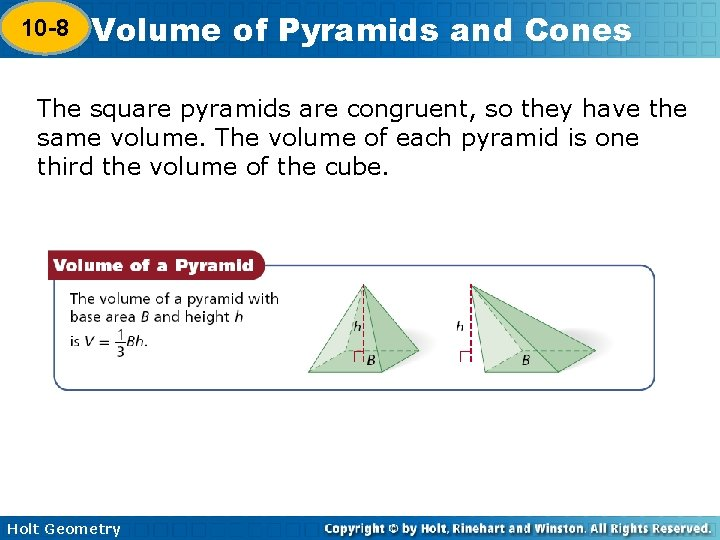10 -8 Volume of Pyramids and Cones 10 -7 The square pyramids are congruent, so they have the same volume. The volume of each pyramid is one third the volume of the cube. Holt Geometry10 -8 Volume of Pyramids and Cones 10 -7 Example 1 A: Finding Volumes of Pyramids Find the volume a rectangular pyramid with length 11 m, width 18 m, and height 23 m. Holt Geometry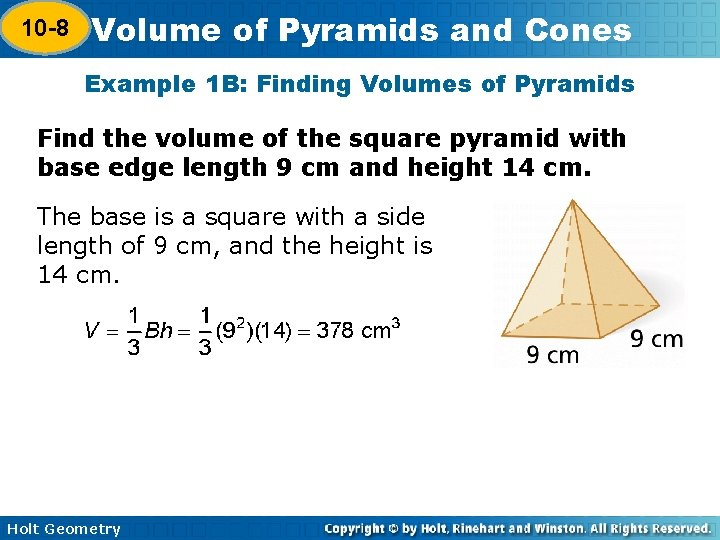10 -8 Volume of Pyramids and Cones 10 -7 Example 1 B: Finding Volumes of Pyramids Find the volume of the square pyramid with base edge length 9 cm and height 14 cm. The base is a square with a side length of 9 cm, and the height is 14 cm. Holt Geometry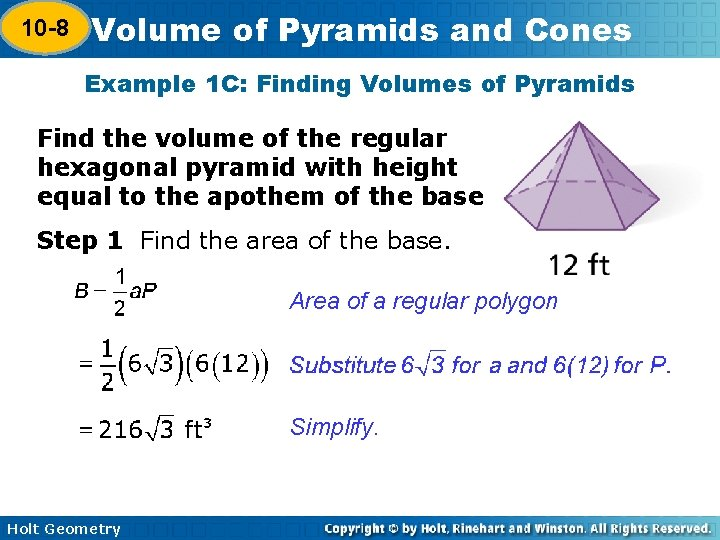10 -8 Volume of Pyramids and Cones 10 -7 Example 1 C: Finding Volumes of Pyramids Find the volume of the regular hexagonal pyramid with height equal to the apothem of the base Step 1 Find the area of the base. Area of a regular polygon Simplify. Holt Geometry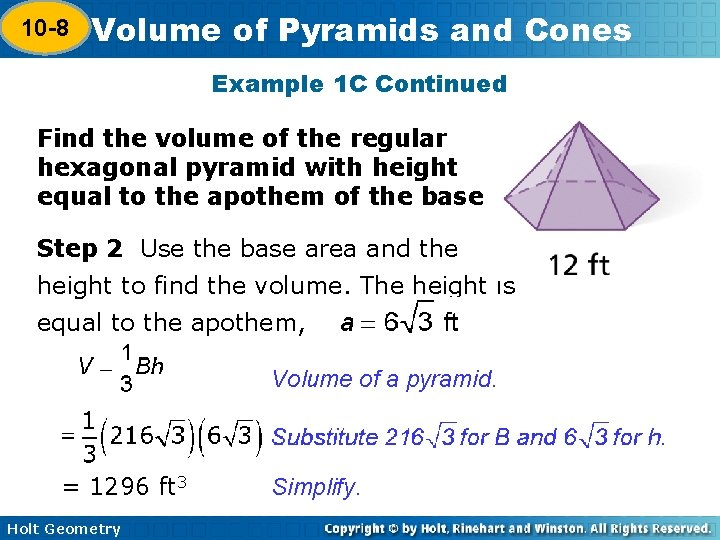10 -8 Volume of Pyramids and Cones 10 -7 Example 1 C Continued Find the volume of the regular hexagonal pyramid with height equal to the apothem of the base Step 2 Use the base area and the height to find the volume. The height is equal to the apothem, . Volume of a pyramid. = 1296 ft 3 Holt Geometry Simplify.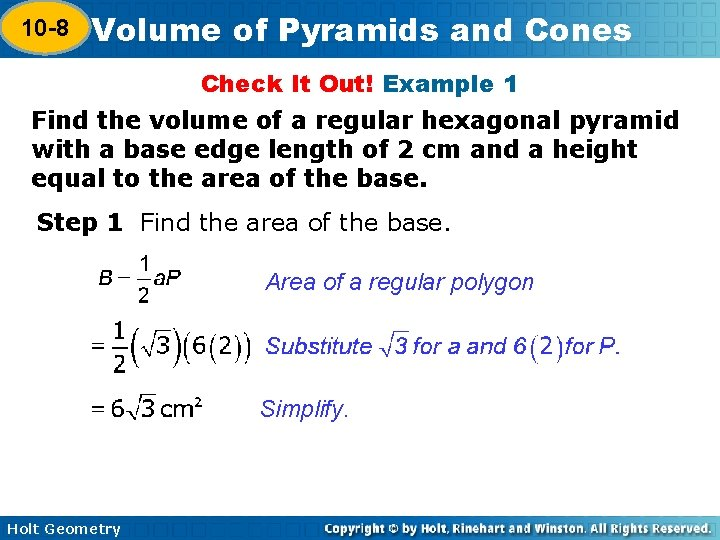10 -8 Volume of Pyramids and Cones 10 -7 Check It Out! Example 1 Find the volume of a regular hexagonal pyramid with a base edge length of 2 cm and a height equal to the area of the base. Step 1 Find the area of the base. Area of a regular polygon Simplify. Holt Geometry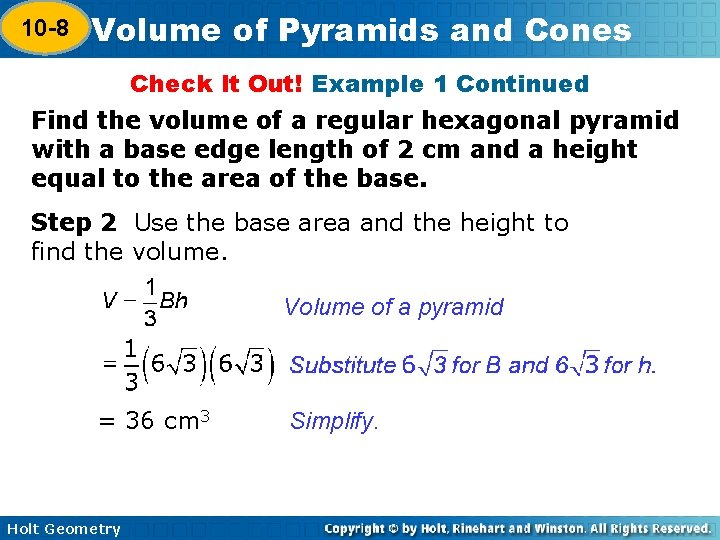10 -8 Volume of Pyramids and Cones 10 -7 Check It Out! Example 1 Continued Find the volume of a regular hexagonal pyramid with a base edge length of 2 cm and a height equal to the area of the base. Step 2 Use the base area and the height to find the volume. Volume of a pyramid = 36 cm 3 Holt Geometry Simplify.10 -8 Volume of Pyramids and Cones 10 -7 Example 2: Architecture Application An art gallery is a 6 -story square pyramid with base area acre (1 acre = 4840 yd 2, 1 story ≈ 10 ft). Estimate the volume in cubic yards and cubic feet. The base is a square with an area of about 2420 yd 2. The base edge length is. The height is about 6(10) = 60 ft or about 20 yd. First find the volume in cubic yards. Volume of a pyramid Holt Geometry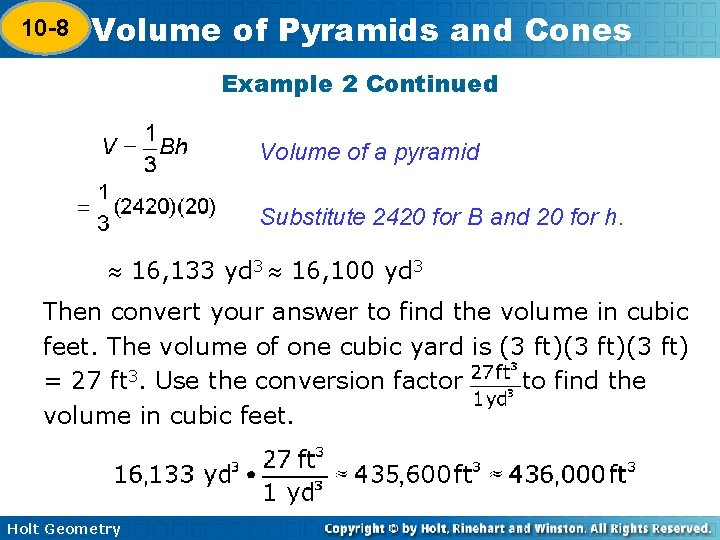10 -8 Volume of Pyramids and Cones 10 -7 Example 2 Continued Volume of a pyramid Substitute 2420 for B and 20 for h. 16, 133 yd 3 16, 100 yd 3 Then convert your answer to find the volume in cubic feet. The volume of one cubic yard is (3 ft)(3 ft) = 27 ft 3. Use the conversion factor to find the volume in cubic feet. Holt Geometry10 -8 Volume of Pyramids and Cones 10 -7 Check It Out! Example 2 What if…? What would be the volume of the Rainforest Pyramid if the height were doubled? Volume of a pyramid. Substitute 70 for B and 66 for h. = 107, 800 yd 3 or 107, 800(27) = 2, 910, 600 ft 3 Holt Geometry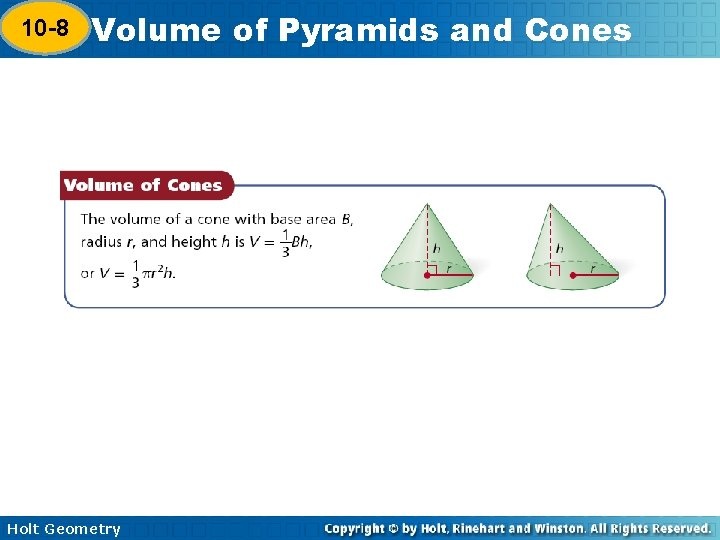10 -8 Volume of Pyramids and Cones 10 -7 Holt Geometry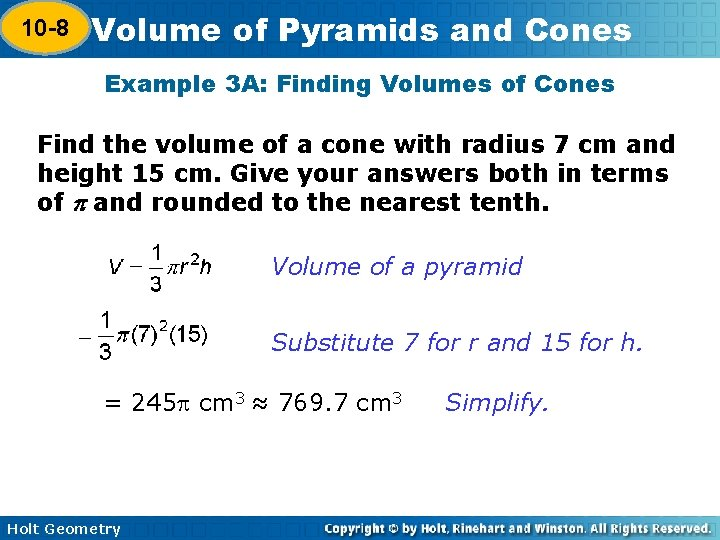10 -8 Volume of Pyramids and Cones 10 -7 Example 3 A: Finding Volumes of Cones Find the volume of a cone with radius 7 cm and height 15 cm. Give your answers both in terms of and rounded to the nearest tenth. Volume of a pyramid Substitute 7 for r and 15 for h. = 245 cm 3 ≈ 769. 7 cm 3 Holt Geometry Simplify.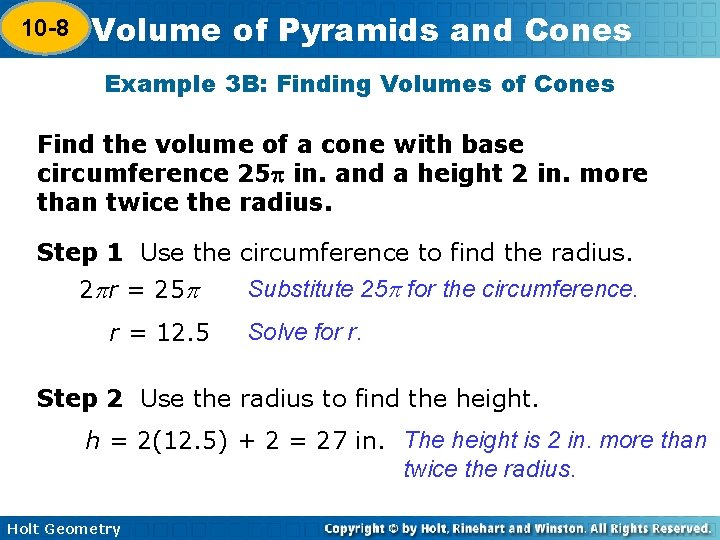10 -8 Volume of Pyramids and Cones 10 -7 Example 3 B: Finding Volumes of Cones Find the volume of a cone with base circumference 25 in. and a height 2 in. more than twice the radius. Step 1 Use the circumference to find the radius. Substitute 25 for the circumference. 2 r = 25 r = 12. 5 Solve for r. Step 2 Use the radius to find the height. h = 2(12. 5) + 2 = 27 in. The height is 2 in. more than twice the radius. Holt Geometry10 -8 Volume of Pyramids and Cones 10 -7 Example 3 B Continued Find the volume of a cone with base circumference 25 in. and a height 2 in. more than twice the radius. Step 3 Use the radius and height to find the volume. Volume of a pyramid. Substitute 12. 5 for r and 27 for h. = 1406. 25 in 3 ≈ 4417. 9 in 3 Holt Geometry Simplify.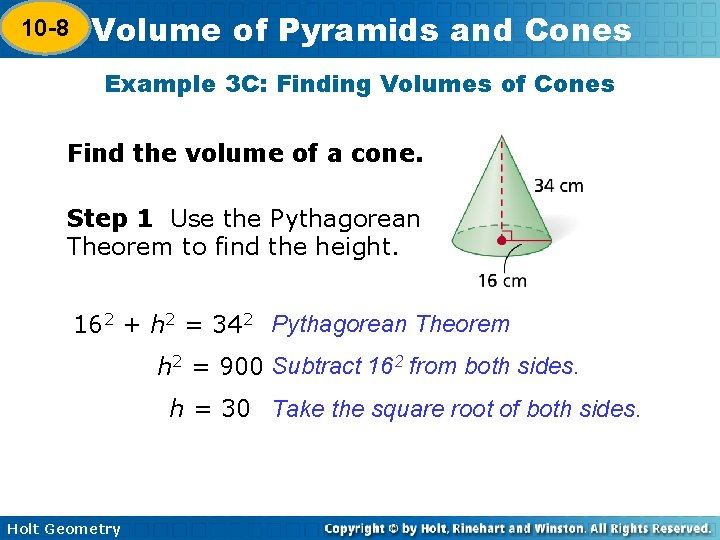10 -8 Volume of Pyramids and Cones 10 -7 Example 3 C: Finding Volumes of Cones Find the volume of a cone. Step 1 Use the Pythagorean Theorem to find the height. 162 + h 2 = 342 Pythagorean Theorem h 2 = 900 Subtract 162 from both sides. h = 30 Take the square root of both sides. Holt Geometry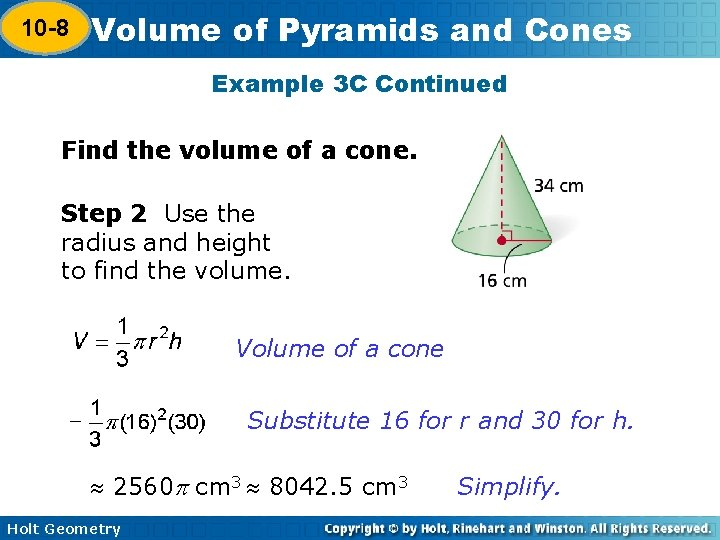10 -8 Volume of Pyramids and Cones 10 -7 Example 3 C Continued Find the volume of a cone. Step 2 Use the radius and height to find the volume. Volume of a cone Substitute 16 for r and 30 for h. 2560 cm 3 8042. 5 cm 3 Holt Geometry Simplify.10 -8 Volume of Pyramids and Cones 10 -7 Check It Out! Example 3 Find the volume of the cone. Volume of a cone Substitute 9 for r and 8 for h. ≈ 216 m 3 ≈ 678. 6 m 3 Simplify. Holt Geometry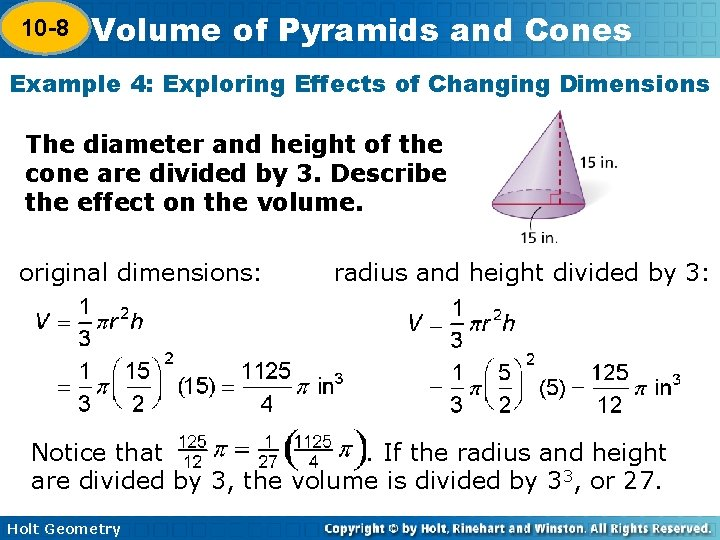10 -8 Volume of Pyramids and Cones 10 -7 Example 4: Exploring Effects of Changing Dimensions The diameter and height of the cone are divided by 3. Describe the effect on the volume. original dimensions: radius and height divided by 3: Notice that. If the radius and height are divided by 3, the volume is divided by 33, or 27. Holt Geometry10 -8 Volume of Pyramids and Cones 10 -7 Check It Out! Example 4 The radius and height of the cone are doubled. Describe the effect on the volume. original dimensions: radius and height doubled: The volume is multiplied by 8. Holt Geometry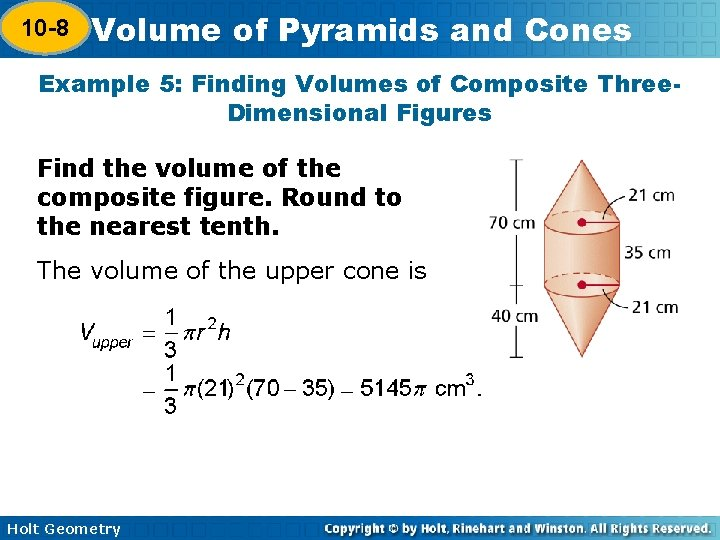10 -8 Volume of Pyramids and Cones 10 -7 Example 5: Finding Volumes of Composite Three. Dimensional Figures Find the volume of the composite figure. Round to the nearest tenth. The volume of the upper cone is Holt Geometry10 -8 Volume of Pyramids and Cones 10 -7 Example 5: Finding Volumes of Composite Three. Dimensional Figures Find the volume of the composite figure. Round to the nearest tenth. The volume of the cylinder is Vcylinder = r 2 h = (21)2(35)=15, 435 cm 3. The volume of the lower cone is The volume of the figure is the sum of the volumes. V = 5145 + 15, 435 + 5, 880 = 26, 460 83, 126. 5 cm 3 Holt Geometry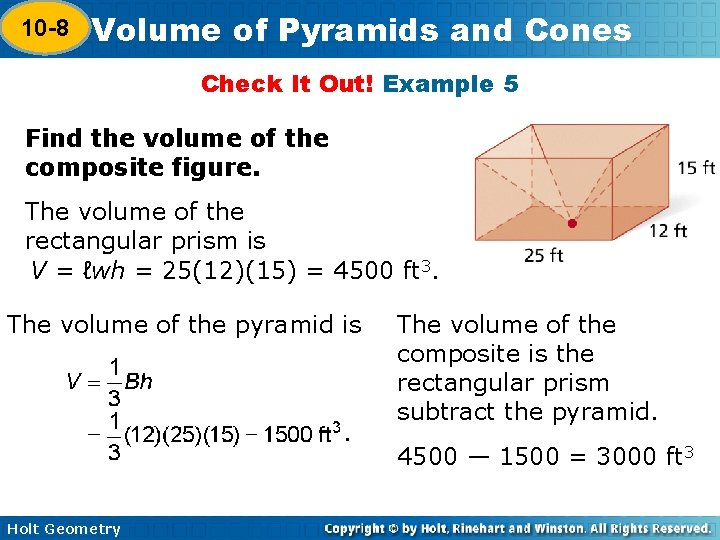10 -8 Volume of Pyramids and Cones 10 -7 Check It Out! Example 5 Find the volume of the composite figure. The volume of the rectangular prism is V = ℓwh = 25(12)(15) = 4500 ft 3. The volume of the pyramid is The volume of the composite is the rectangular prism subtract the pyramid. 4500 — 1500 = 3000 ft 3 Holt Geometry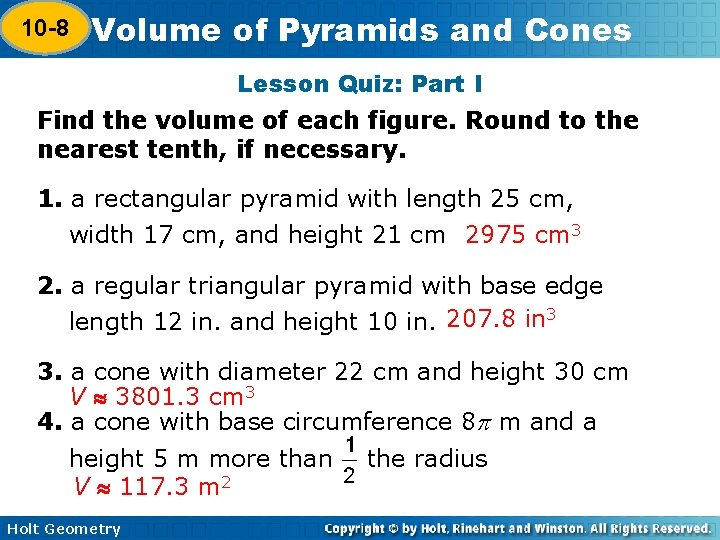10 -8 Volume of Pyramids and Cones 10 -7 Lesson Quiz: Part I Find the volume of each figure. Round to the nearest tenth, if necessary. 1. a rectangular pyramid with length 25 cm, width 17 cm, and height 21 cm 2975 cm 3 2. a regular triangular pyramid with base edge length 12 in. and height 10 in. 207. 8 in 3 3. a cone with diameter 22 cm and height 30 cm V 3801. 3 cm 3 4. a cone with base circumference 8 m and a height 5 m more than V 117. 3 m 2 Holt Geometry the radius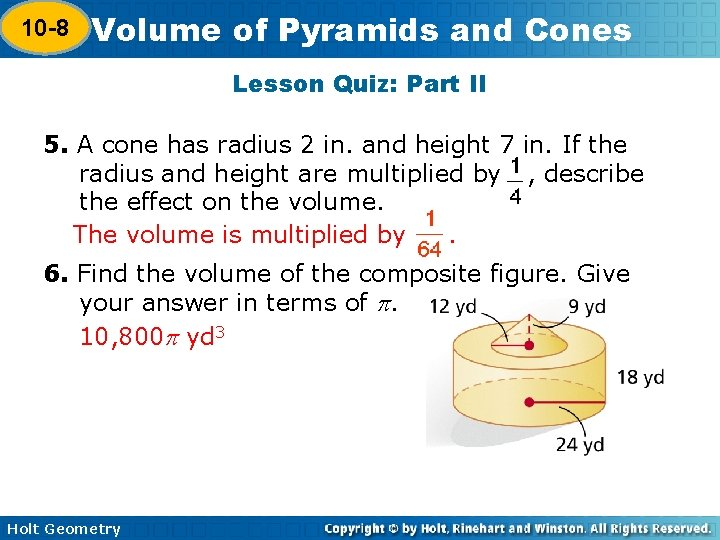10 -8 Volume of Pyramids and Cones 10 -7 Lesson Quiz: Part II 5. A cone has radius 2 in. and height 7 in. If the radius and height are multiplied by , describe the effect on the volume. The volume is multiplied by. 6. Find the volume of the composite figure. Give your answer in terms of . 10, 800 yd 3 Holt Geometry